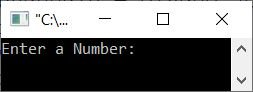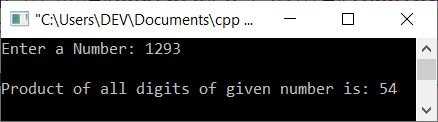# C++ Program to Find and Print the Product of the Digits of a Number

This article provides some programs in C++ that find and print the product of the digits of a number entered by the user at run-time. The program is created in the following ways:

And at last, one extra program is also included that finds the product of the only non-zero digit of a given number.

## Using a while loop, calculate the product of a number's digits

The question is: write a C++ program that receives a number from the user and prints the product or multiplication of its digit. The program given below is its answer:

```#include<iostream>

using namespace std;
int main()
{
int num, rem, prod=1;
cout<<"Enter a Number: ";
cin>>num;
while(num>0)
{
rem = num%10;
prod = prod*rem;
num = num/10;
}
cout<<"\nProduct of all digits of given number is: "<<prod;
cout<<endl;
return 0;
}```

The initial output produced by the above C++ program on finding and printing the product of all digits of a given number is given in the following snapshot:Now supply the input, say 1293 as a number, and press the ENTER key to print the product of all four digits as shown in the snapshot given here:The dry run of the following block of code from the above program:

```while(num>0)
{
rem = num%10;
prod = prod*rem;
num = num/10;
}```

with prod=1 and num=1293, goes like this:

• The condition num>0 or 1293>0 is satisfied.
• Therefore, program flow goes inside the loop, and all three statements get executed one by one.
• That is, num%10 or 1293%10 or 3 gets initialized to rem, prod*rem or 1*3 or 3 gets initialized to prod, and num/10 or 1293/10 or 129 gets initialized to num.
• Now the condition of the loop gets evaluated again with the new value of num.
• That is, the condition num>0 or 129>0 evaluates to true once more.
• Therefore, program flow again goes inside the loop.
• This process continues until the condition is evaluated as false.
• In this way, the product of the digits of a number gets calculated.

## Find the Product of a Number's Digits Using a for Loop

This program performs the same function as the previous one, but it was written in a for loop rather than a while loop.

```#include<iostream>

using namespace std;
int main()
{
int num, rem, prod;
cout<<"Enter a Number: ";
cin>>num;
for(prod=1; num>0; num=num/10)
{
rem = num%10;
prod = prod*rem;
}
cout<<"\nProduct of all digits of given number is: "<<prod;
cout<<endl;
return 0;
}```

In the above program, the following two statements:

```rem = num%10;
prod = prod*rem;```

can also be replaced with the single statement given below:

`prod = prod*(num%10);`

## Find the product of the digits (except 0) of a number

Since both the programs given above give 0 as output if any digit of the given number is 0, let's modify and create another program that finds the product of only one non-zero digit of the given number:

```#include<iostream>

using namespace std;
int main()
{
int num, rem, prod;
cout<<"Enter a Number: ";
cin>>num;
for(prod=1; num>0; num=num/10)
{
rem = num%10;
if(rem>0)
prod = prod*rem;
}
cout<<"\nProduct of all digits of given number is: "<<prod;
cout<<endl;
return 0;
}```

This program produces the same output as the previous program. But the previous program printed 0 if any digit of the given number was equal to 0, whereas this program does not print 0 if any digit of the given number is 0. Rather, this only multiplies non-zero digits and prints the multiplication result.

#### Modified Version of the Previous Program

This program is created in a way to provide a good user interface by showing the ongoing multiplication of digits like 3*2*4.

```#include<iostream>

using namespace std;
int main()
{
int num, rem, prod=1;
cout<<"Enter a Number: ";
cin>>num;
if(num>0)
cout<<endl;
else
{
cout<<"\nThe number must be greater than 0!\n";
return 0;
}
while(num>0)
{
rem = num%10;
num /= 10;
if(rem>0)
{
prod = prod*rem;
if(num>0)
cout<<rem<<"*";
else
cout<<rem;
}
}
cout<<" = "<<prod;
cout<<endl;
return 0;
}```

This program produces the following output when the user enters a number, say 12032, as input:You're seeing the digit in the form 2*3*2*1 (in reverse order). because while finding the remainder using rem = num%10, it gives the last digit. But you can reverse the number first, then do the task.

To do it, put the reverse of a number code in the very first if's body and initialize the reversed result to the number num itself, then proceed. Come on, do it yourself for practice.

C++ Quiz

« Previous Program Next Program »